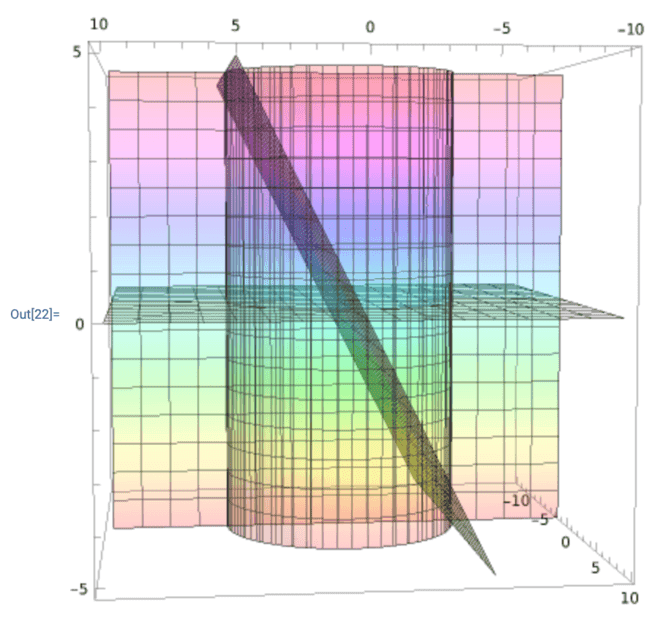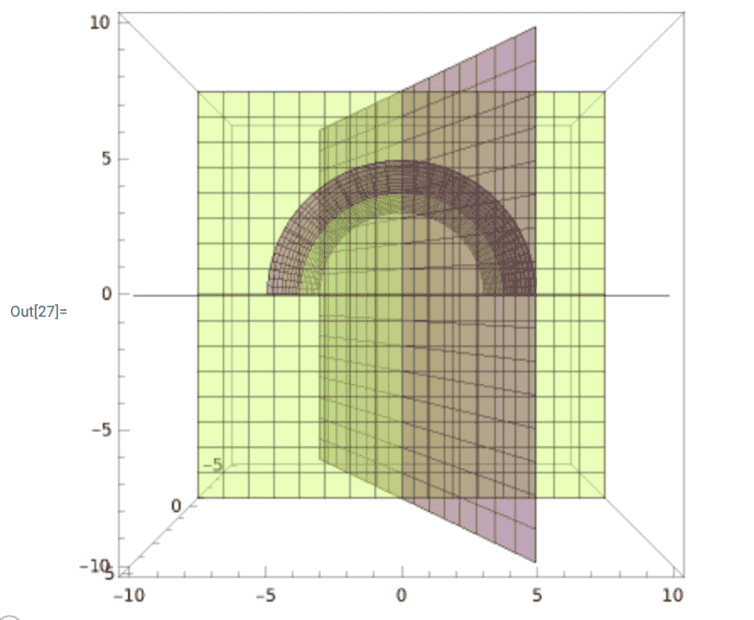# Volume of solid region double integral

• woopydalan
In summary: No, f(x,y)=x does not make z=x. f(x,y) is given as a bounding surface of z, not the value of z everywhere. We can rewrite the conditions as"where z lies between the values 0 and x".Personally I've never been happy with the presumption that areas and volumes cannot be negative, but @Delta2 is right that that is the usual convention, so the correct volume integral is ##\int|z|dxdy##,...

#### woopydalan

Homework Statement
Set up the integral for both orders of integration and use the more convenient order to find the volume of the solid region bounded by the surface f(x, y) and the planes.
##f(x, y) = x##
Planes: ##z = 0##, ##y = \sqrt{25 − x^2}##, ##y = 0##
Relevant Equations
##\iint\limits_R f(x,y) \, dA##
I sketched this out. With the z=0 and y=0 boundaries, we are looking at ##z \geq 0## and ##y \geq 0##

I believe ##0 \leq x \leq 5## because of the boundary of ##y=\sqrt{25-x^2}##.

This is my region

##\int_0^5 \int_0^\sqrt{25-x^2} x \, dydx ##
## =\int_0^5 xy \vert_{0}^{\sqrt{25-x^2}} \, dx##
## =\int_0^5 x\sqrt{25-x^2} \, dx##
Using U-substitution ##u = 25-x^2##, and ##du = -2x \, dx##,
## =-\frac{1}{2} \int_0^5 u^{\frac{1}{2}} \, du##
## =-\frac{1}{2} \cdot \frac{2}{3} u^{\frac{3}{2}} \vert_{0}^{5}##
##=\frac{125}{3}##

However, it seems this is not correct as when I submit this answer I get a response that it is incorrect. Am I doing something wrong with my boundaries for the integrals?

Last edited:
woopydalan said:
Using U-substitution
So what are the bounds on ##u## ?

PS you are also sweeping a minus sign under the rug ...

PPS##\qquad##!​

##\ ##

Ok sorry, I didn't write that correctly in the post, but it would be

##-\frac{1}{2} \cdot \frac{2}{3} (25-x^2)^{\frac{3}{2}} \vert_{0}^{5} ##
## = 0 - (-\frac{1}{3} \cdot 25^{\frac{3}{2}}) ##
## = \frac{125}{3} ##

In these problems, the challenge is usually setting up the bounds for the integrals. I'm wondering if I chose the incorrect ones? It's easy enough to verify the integral solution with wolframalpha.

Oops, sorry I overlooked that. In that case I don't have any other suggestions. And the numerial outcome doesn't look all that weird either. @haruspex, any idea ?

##\ ##

What makes you think x=0 is a boundary?

•BvU and Delta2
haruspex said:
What makes you think x=0 is a boundary?
Well, as I explained in the OP, because ##y = \sqrt{25-x^2}##, so certainly ##x=5## is a boundary. If not ##x=0##, then ##x=-5##. If it's -5, then the volume is 0.

•Delta2
yes I also believe x=-5 is the correct boundary, but since you want the volume you should calculate $$\iint |f(x,y)|dxdy$$

Delta2 said:
yes I also believe x=-5 is the correct boundary, but since you want the volume you should calculate $$\iint |f(x,y)|dxdy$$
Interesting, that was never in the textbook as far as the absolute value.

woopydalan said:
Interesting, that was never in the textbook as far as the absolute value.
Well, if you don't put the absolute value and you go ahead calculate the integral without it and with the new x-boundaries (from -5 to +5) you ll get zero as result.

Delta2 said:
Well, if you don't put the absolute value and you go ahead calculate the integral without it and with the new x-boundaries (from -5 to +5) you ll get zero as result.
Understood, that's why I figured the boundary is x=0.

Nope x=0 is not the correct boundary, it is x=-5 to x=+5 and the actual volume is double of what you calculated...

Delta2 said:
Nope x=0 is not the correct boundary, it is x=-5 to x=+5 and the actual volume is double of what you calculated...
Ok, I got it. But I wonder if ##\lvert f(x,y) \rvert## is a general rule, or just because the nature of this function is why it was necessary to use

In my opinion it is a general rule if you want to calculate the volume between some region of the xy-plane (where z=0), and z=f(x,y), otherwise if you don't put the absolute value, you ll end up subtracting volume in the region of xy-plane where f(x,y) becomes negative. If f(x,y) is positive for all x,y in the region of interest in the xy-plane , then ok there is no need of absolute value.

Delta2 said:
In my opinion it is a general rule if you want to calculate the volume between some region of the xy-plane (where z=0), and z=f(x,y), otherwise if you don't put the absolute value, you ll end up subtracting volume in the region of xy-plane where f(x,y) becomes negative. If f(x,y) is positive for all x,y in the region of interest in the xy-plane , then ok there is no need of absolute value.
Thinking about it a little more now, how can x < 0? The boundary z=0, and if x=z, then you shouldn't have a negative value for x, no?

Imagine the solid shape is made of wood. Two parts. ##|f| ## to get the volume.

##\ ##

•Leo Liu
BvU said:
Imagine the solid shape is made of wood. Two parts. ##|f| ## to get the volume.

##\ ##
I don't follow that at all. What about the boundary z=0? So if f(x,y) = z = x, how can x<0?

What's wrong with the volume enclosed by ##f,\ \ y=0,\ \ z=0 \ ## for ##x<0\ ##?
(as @haruspex mentioned)
It satisfies all the criteria just as well as the part with ##x>0## !

##\ ##

woopydalan said:
I don't follow that at all. What about the boundary z=0? So if f(x,y) = z = x, how can x<0?
No, f(x,y)=x does not make z=x. f(x,y) is given as a bounding surface of z, not the value of z everywhere. We can rewrite the conditions as"where z lies between the values 0 and x".
Personally I've never been happy with the presumption that areas and volumes cannot be negative, but @Delta2 is right that that is the usual convention, so the correct volume integral is ##\int|z|dxdy##, with the ranges always running from the lower bound to the higher.

•Delta2
haruspex said:
No, f(x,y)=x does not make z=x. f(x,y) is given as a bounding surface of z, not the value of z everywhere. We can rewrite the conditions as"where z lies between the values 0 and x".
Personally I've never been happy with the presumption that areas and volumes cannot be negative, but @Delta2 is right that that is the usual convention, so the correct volume integral is ##\int|z|dxdy##, with the ranges always running from the lower bound to the higher.
I guess I don't understand. ##f(x,y) = z## is the 3-dimensional equivalent of ##f(x) = y##. If the surface is bound by ##z=0## and ##y= \sqrt {25-x^2}##, and ##y=0##, I know that ##y \geq 0##. How can ##f(x,y) < 0##? If that's the case, I don't really even know what the bound ##z=0## means or why it matters if ##z < 0## is permissible.

The bounds of z=0 and z=f(x,y), means that our volume of interest contains points (x,y,z) such that $$0\leq z\leq f(x,y)$$, OR $$f(x,y)\leq z\leq 0$$ (and the other constraints that refer to x and y). So the bound of z=0 doesn't mean necessarily that it is a lower bound but it can be an upper bound as well IF f(x,y) goes negative.

Last edited:
woopydalan said:
I guess I don't understand. ##f(x,y) = z## is the 3-dimensional equivalent of ##f(x) = y##. If the surface is bound by ##z=0## and ##y= \sqrt {25-x^2}##, and ##y=0##, I know that ##y \geq 0##. How can ##f(x,y) < 0##? If that's the case, I don't really even know what the bound ##z=0## means or why it matters if ##z < 0## is permissible.
It might help if you can visualise it.
##y= \sqrt {25-x^2}## Is a horizontal semicircle radius 5, centred at the origin. In 3D, that as a boundary, combined with the y=0 boundary, defines an infinite vertical half cylinder.
z=0 is the horizontal plane through the origin, while z=x is a plane at 45 degrees containing the y axis.
These three boundaries enclose two separate wedges meeting at the y axis.
In one wedge, the z=0 plane is the upper bound, while in the other wedge it is the lower bound.

•Delta2
So basically z can be anything EXCEPT 0. Kind of a dumb boundary IMO

woopydalan said:
So basically z can be anything EXCEPT 0. Kind of a dumb boundary IMO
It's no different in that respect to the way the other boundaries are prescribed. You are given that ##y=\sqrt{25-x^2}## is a boundary, not that ##y<\sqrt{25-x^2}##. Which side of that lies the enclosed region has to be deduced from the other boundaries. The only complication in the z=0 boundary is that the enclosed region lies a part on each side.

haruspex said:
It might help if you can visualise it.Alternatively, you can use integration under polar coordinates:
$$\int_0^{\pi}\int_0^5 | r\cos \theta \, r | drd\theta$$

#### Attachments

Last edited:
•Delta2
Leo Liu said:
View attachment 286087
View attachment 286088
View attachment 286089
Alternatively, you can use integration under polar coordinates:
$$\int_0^{\pi/2}\int_0^5 r\sin \theta \, rdrd\theta$$
Nice diagrams, thanks, except that as noted earlier in the thread the enclosed volume includes an identical region with z<0 and x<0.
For the region shown in your diagrams, the volume was found in post #1.

haruspex said:
Nice diagrams, thanks, except that as noted earlier in the thread the enclosed volume includes an identical region with z<0 and x<0.
For the region shown in your diagrams, the volume was found in post #1.
Thanks for pointing out my mistake :D Do they look good now? Also the bounds for theta are [0, Pi] under polar coordinates right?

Leo Liu said:
Thanks for pointing out my mistake :D Do they look good now? Also the bounds for theta are [0, Pi] under polar coordinates right?
Yes, and yes.

woopydalan said:
So basically z can be anything EXCEPT 0. Kind of a dumb boundary IMO
z can be anything between 0 and f(x,y). But don't take for granted that f(x,y) is positive.